Courses
Courses for Kids
Free study material
Offline Centres
More

# 2 Digit Addition with RegroupingLast updated date: 04th Dec 2023
Total views: 102.6k
Views today: 1.02k## Introduction

The addition is a process of combining two or more numbers to obtain their sum. When the total of the values in one place value column exceeds nine, regrouping is employed. No matter how many numbers are included in the query or how many digits each number has, this method of moving a number to the next place value column can be applied with any addition. Setting the table, getting change at the store, playing some games, and many other daily tasks all benefit from the addition.

By keeping in mind the place value of numbers, 2-digit addition can be performed. Ones and tens are used to express the place value of 2-digit numerals. We organise the integers column-wise beneath the corresponding place values when adding two digits together.

You can mentally add two numbers by following some simple instructions.

The digits can be added one at a time using this procedure. You can perform 2-digit addition both with and without regrouping.

For instance, let’s add 57 and 31## 2 Digit Addition without Regrouping:

A 2-digit addition without regrouping is the simplest addition. This method is employed in daily life while carrying out any kind of financial transaction. When the sum is less than or equal to 9, we write the number in the appropriate place value column and do not carry any numbers to the next column.

## 2 Digit Addition with Regrouping:

We can perform 2-digit addition by regrouping the given numbers as a result of a situation. If the sum of the numbers in one column is greater than 9, we must move the excess number to the following column. We start in the ones column and go left toward the tens column when regrouping two-digit values.

Let's use an illustration to better grasp this: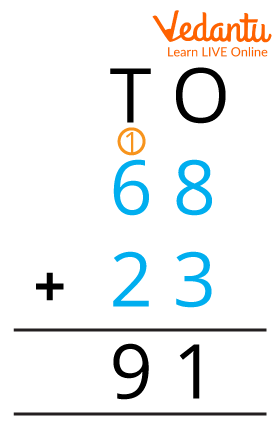## Steps for Doing 2 Digit Addition with regrouping:

Let us understand it with one example :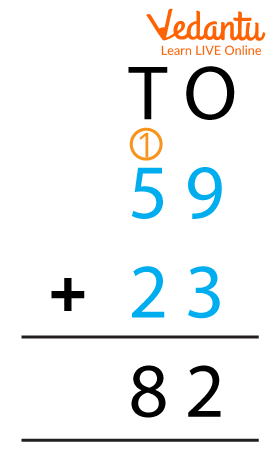STEP 1: Sort the numbers into columns based on their place value, such as ones and tens column-wise. As a result, 9 and 3 will go in the ones column and 5 and 2 in the tens column.

STEP 2: Start adding the numbers from the right, i.e., the ones column and proceed towards the left, i.e., the tens column. Here, 9 + 3 = 12.

STEP 3: A double-digit number results when the total of the ones in the column is more than 9. In the case of a double-digit number, the ones digit goes in the ones column, while the tens digit is carried over or regrouped to the tens column. We add 1 to the tens column because 12 is more than 9, and we write 2 as the sum in the ones column because 12 is greater than 9.

STEP 4: We add the value that was carried over from the ones column when adding the tens column. We will get 8 when we add 5 + 2 + 1 (carry-over).

STEP 5: We get the total after adding the values from both columns.

The result of adding both columns is 59 + 23 = 82.

## Properties of 2 Digit Addition with Regrouping:

PROPERTY 1 :

Commutative Property: The commutative property of addition states that no matter how the addends are arranged, the sum remains constant.

PROPERTY 2 :

Identity Property: According to the identity feature of addition, a number remains unchanged when a zero is added to it.

PROPERTY 3 :

Associative Property: When three or more numbers are added, the sum is the same regardless of which two addends are added first, according to the associative property of addition.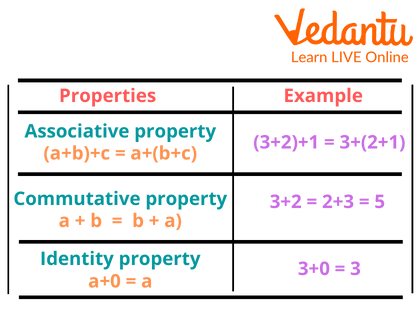## Solved Examples:

Solution: The sum of 58 and 23 is 81.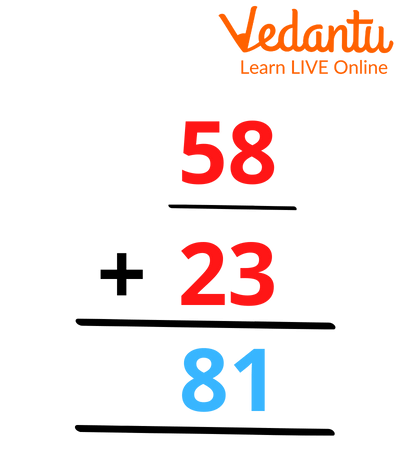2: If Aman has 87 chocolates, his teacher gives him 45 more chocolates. How many chocolates Aman has in total?

Solution: If Aman had 87 chocolates and his teacher gave him 45 more.

Thus,

The number of chocolates Aman have are 87

The number of chocolates the teacher gave is 45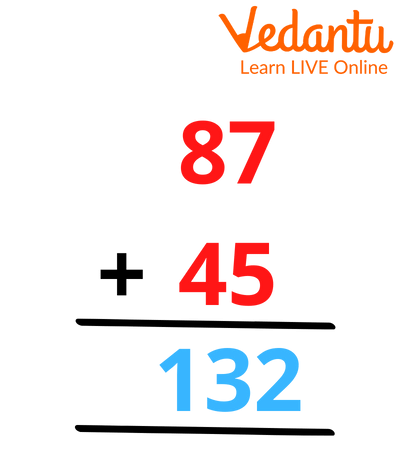In total Aman has 132 chocolates.

3: There are 756 boys and 525 girls in the school. How many children were there altogether?

Solution: Number of boys = 756

Number of girls =+ 525

Total children = 1281

There were 1281 children in the school.

## Conclusion:

When the sum is less than or equal to 9, we regroup the numbers and write the result beneath the appropriate place value column before moving the results to the next column.

## FAQs on 2 Digit Addition with Regrouping

1. What is regrouping in addition?

The addition without regrouping method to combine two or more numbers of any size. It is used in combination with the column addition method, in which integers are added one column at a time and sums are arranged vertically. Regrouping may also be referred to as "carrying over."

2. If we add any number with 0 what will we get?

If we add any number with 0 we will get the same number itself.

3. What will be obtained if we add 1 to any number?

We will be obtaining the successor of that number if we add 1 to it.